# Basics

This section gives some examples to get started quickly creating simple graphs.

## Single Line¶

The only required parameter is `q` which specifies the query expression for a line. The other two common parameters are for setting the start time, `s`, and the end time, `e`, for the data being shown. Usually the start time will be set relative to the end time, such as `e-3h`, which indicates 3 hours before the end time. See time parameters for more details on time ranges.

Putting it all together:

```/api/v1/graph?
e=2012-01-01T00:00
&q=
name,sps,:eq
&s=e-2d
```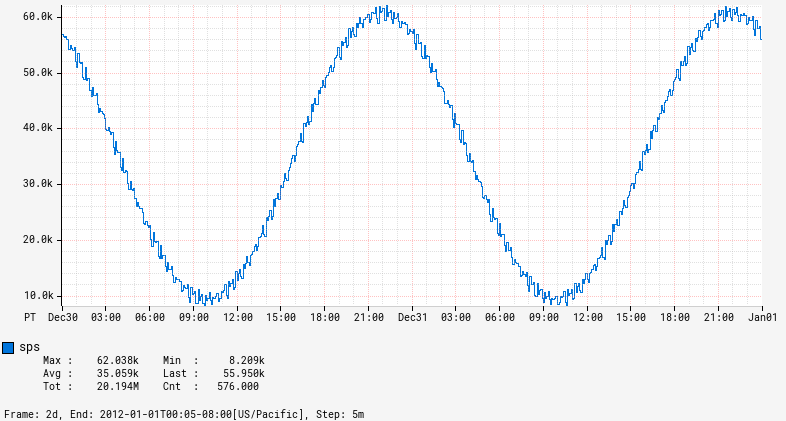The resulting PNG plot displays time along the X axis, automatically scaled to the proper time range. The Y axis labels are scaled using metric prefixes to show the measured value. A legend is displayed under the plot with the name(s) of the expression results and a set of statistics computed on the plotted data for the time window. The small text at the very bottom reflect query parameters and step size along with some processing statistics.

The graph title can be set using the `title` parameter. Similarly, a Y-axis label can be set using the `ylabel` parameter.

```/api/v1/graph?
e=2012-01-01T00:00
&q=
name,sps,:eq
&s=e-2d
&title=Starts+Per+Second
&ylabel=sps
```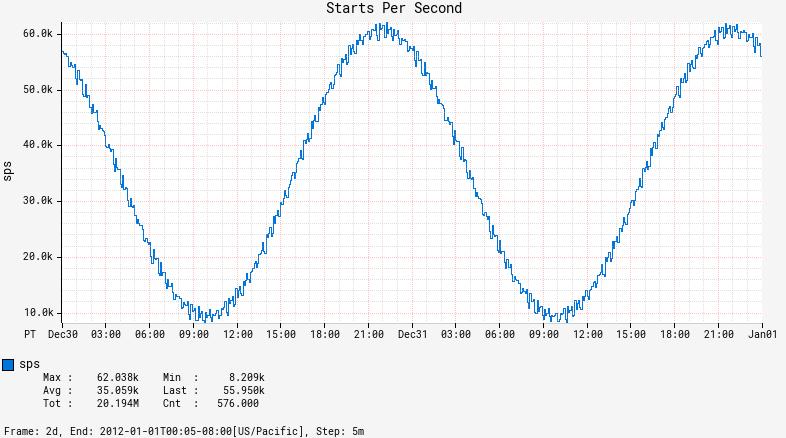## Multiple Lines¶

Multiple expressions can be placed on a chart by concatenating the expressions, e.g., showing a query expression along with a constant value:

```/api/v1/graph?
e=2012-01-01T00:00
&q=
name,sps,:eq,
500e3
&s=e-2d
```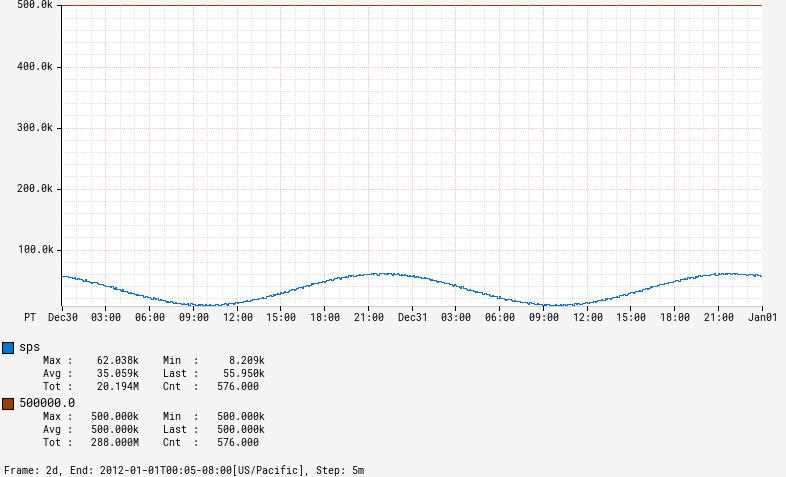## Group By¶

Multiple lines can also be a result of a single expression via group by.

```/api/v1/graph?
e=2012-01-01T00:00
&q=
name,sps,:eq,
(,nf.cluster,),:by
&s=e-2d
```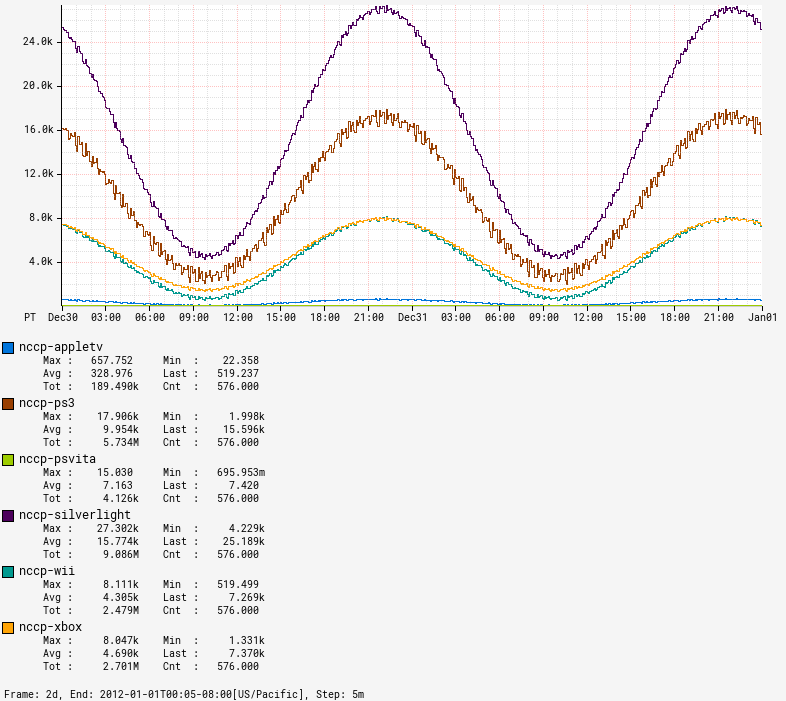## Simple Math¶

A number of operators are provided to manipulate a line. See the math section of the stack language tutorial for a complete list. Example that negates the value of a line:

```/api/v1/graph?
e=2012-01-01T00:00
&q=
name,sps,:eq,
:neg
&s=e-2d
```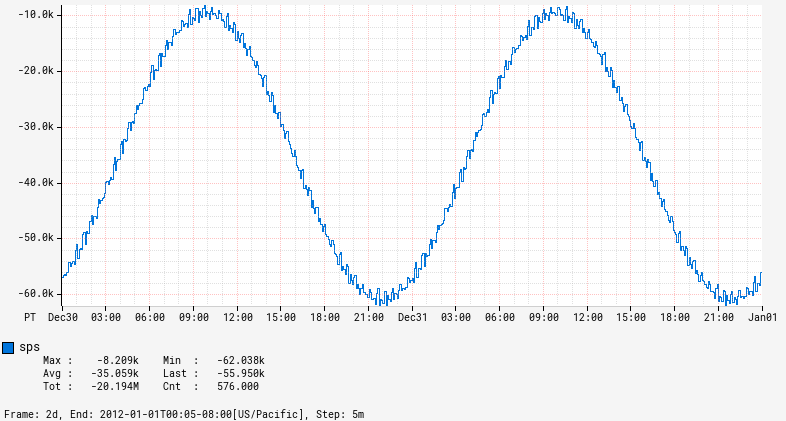Example that negates and then applies absolute value to get the original value back (since all values were positive in the input):

```/api/v1/graph?
e=2012-01-01T00:00
&q=
name,sps,:eq,
:neg,
:abs
&s=e-2d
```## Binary Operations¶

Lines can be combined using binary math operators such as add or multiply. Example using divide:

```/api/v1/graph?
e=2012-01-01T00:00
&q=
name,sps,:eq,
1000,:div
&s=e-2d
```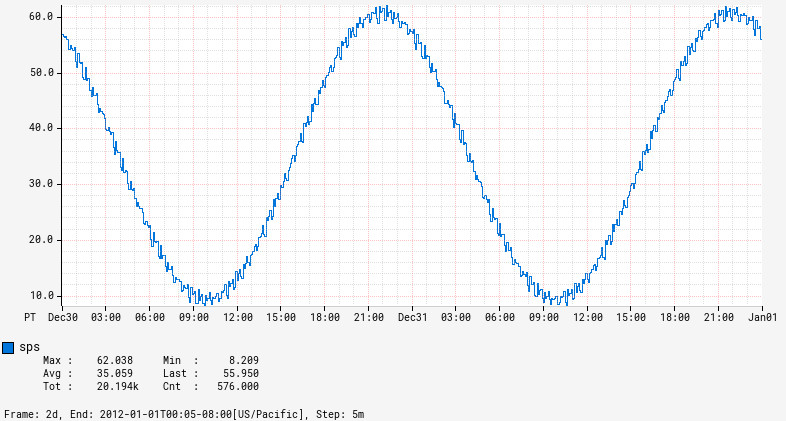If used with a group by, then either:

• Both sides have the same group by clause. In this case an inner join will be preformed and the binary operation will be applied to the corresponding entries from both sides.
• One side is not a grouped expression, and the binary operation will be applied for each instance in the grouped result set.

### Both Sides Grouped¶

Dividing by self with both sides grouped:

```/api/v1/graph?
e=2012-01-01T00:00
&q=
name,sps,:eq,
(,nf.cluster,),:by,
:dup,
:div
&s=e-2d
```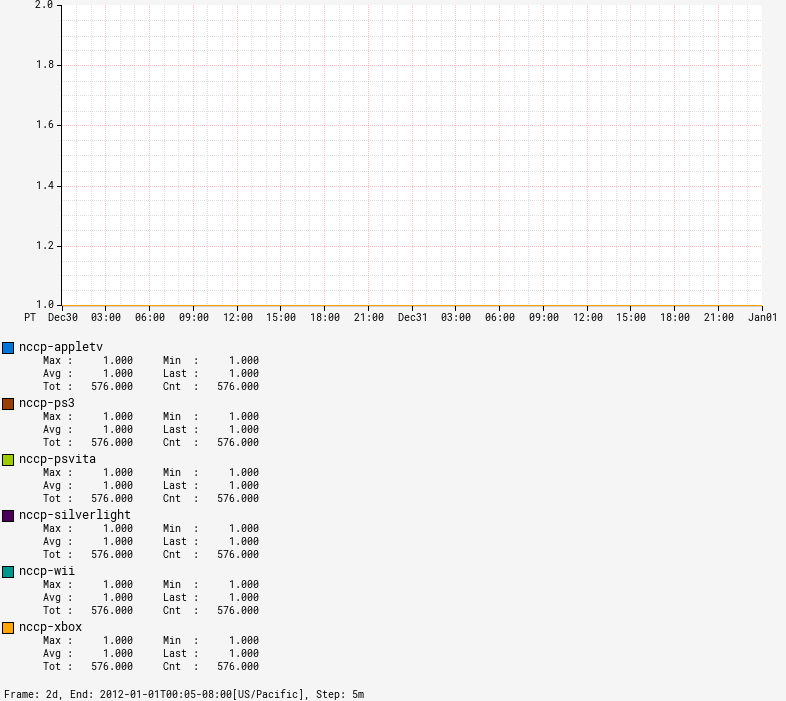### One Side Grouped¶

Dividing a grouped expression by a constant:

```/api/v1/graph?
e=2012-01-01T00:00
&q=
name,sps,:eq,
(,nf.cluster,),:by,
1000,:div
&s=e-2d
```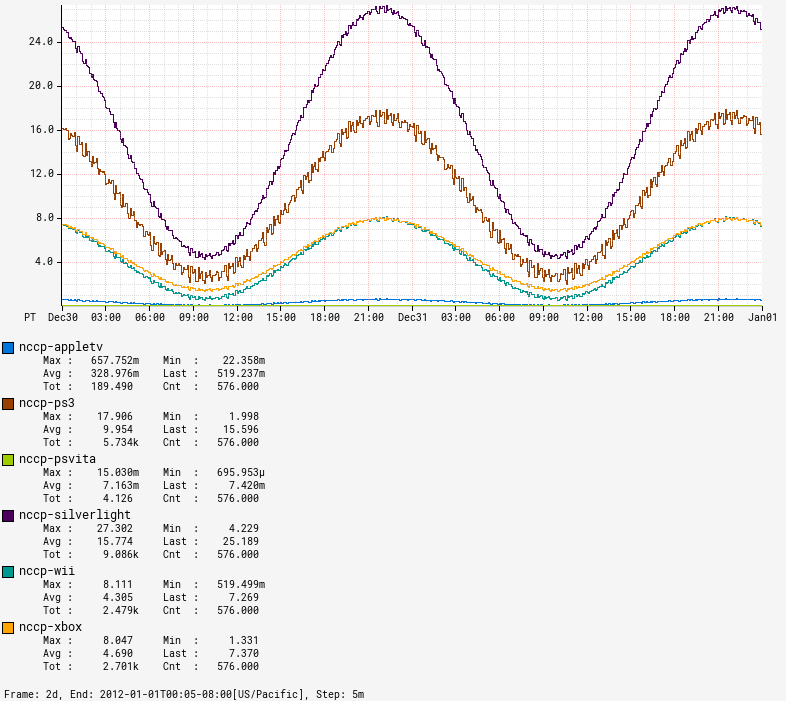Equivalent to the previous expression, but the right-hand side is grouped and it uses multiply instead of divide:

```/api/v1/graph?
e=2012-01-01T00:00
&q=
0.001,name,sps,:eq,
(,nf.cluster,),:by,
:mul
&s=e-2d
```